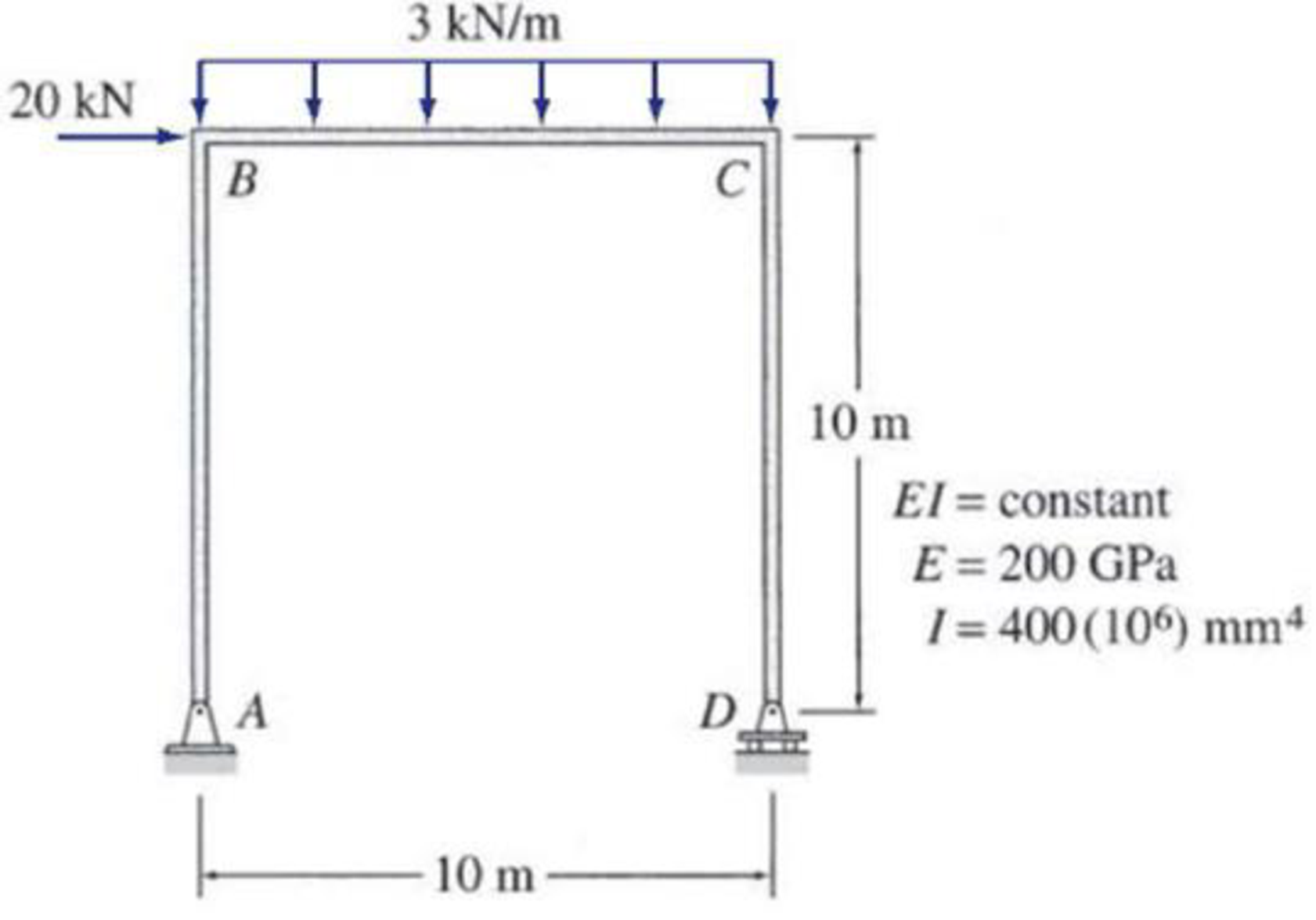# 7.68 and 7.69 Use Castigliano’s second theorem to determine the horizontal deflection at joint C of the frames shown in Figs. P7.45 and P7.46. FIG. P7.45, FIG. P7.68

#### Solutions

Chapter
Section
Chapter 7, Problem 68P
Textbook Problem
166 views

## 7.68 and 7.69 Use Castigliano’s second theorem to determine the horizontal deflection at joint C of the frames shown in Figs. P7.45 and P7.46.FIG. P7.45, FIG. P7.68

To determine

Find the horizontal deflection at joint C of the frame using Castigliano’s second theorem.

### Explanation of Solution

Given information:

The frame is given in the Figure.

The value of E is 200 GPa and I is 400×106mm4.

Apply the sign conventions for calculating reactions, forces and moments using the three equations of equilibrium as shown below.

• For summation of forces along x-direction is equal to zero (Fx=0), consider the forces acting towards right side as positive (+) and the forces acting towards left side as negative ().
• For summation of forces along y-direction is equal to zero (Fy=0), consider the upward force as positive (+) and the downward force as negative ().
• For summation of moment about a point is equal to zero (Matapoint=0), consider the clockwise moment as negative and the counter clockwise moment as positive.

Calculation:

Let apply a fictitious load P at joint C in the horizontal direction to find the deflection.

The value of fictitious load P is zero.

Sketch the frame with fictitious load P as shown in Figure 1.

Let the equation for bending moment at distance x in terms of load P be M, the derivative of M with respect to P is MP.

Find the reactions at the supports A and D:

Summation of moments about A is equal to 0.

MA=0Dy(10)3(10)(102)20(10)P(10)=0Dy=35+P

Summation of forces along y-direction is equal to 0.

+Fy=0Ay+Dy3(10)=0Ay+35+P3(10)=0Ay=5+P

Summation of forces along x-direction is equal to 0.

+Fx=0Ax+20+P=0Ax=20+P

Find the equations for M and MP for the 2 segments of the frame as shown in Table 1.

 Segment x-coordinate M ∂M∂P Origin Limits (m) AB A 0−10 (20+P)x x CB C 0−10 (35+P)x−1.5x2 x

The expression for horizontal deflection at C using Castigliano’s second theorem (ΔC) is shown as follows:

ΔC=0L(MP)MEIdx (1)

Here, L is the length of the beam.

Rearrange Equation (1) for the limits 010 and 010 as follows.

ΔC=1EI[010(MP)Mdx+010(MP)Mdx]

Substitute the value of fictitious load P as zero in the Column 4 of Table 1

### Still sussing out bartleby?

Check out a sample textbook solution.

See a sample solution

#### The Solution to Your Study Problems

Bartleby provides explanations to thousands of textbook problems written by our experts, many with advanced degrees!

Get Started

Find more solutions based on key concepts
Name at least five different materials that are used in a refrigerator.

Engineering Fundamentals: An Introduction to Engineering (MindTap Course List)

What is a common problem in calculating start and finish times? Provide an example.

Systems Analysis and Design (Shelly Cashman Series) (MindTap Course List)

Suspicious File Attachment You receive an email message that appears to be from someone you know. When you try ...

Enhanced Discovering Computers 2017 (Shelly Cashman Series) (MindTap Course List)

Convert 5 megawatts of power into BTU/hr, ft-lbs/s, and kJ/hr.

Fundamentals of Chemical Engineering Thermodynamics (MindTap Course List)

Describe two safety precautions you should observe when using layout dye.

Precision Machining Technology (MindTap Course List)

What is a stored procedure, and why is it particularly useful? Give an example.

Database Systems: Design, Implementation, & Management

If your motherboard supports ECC DDR3 memory, can you substitute non-ECC DDR3 memory?

A+ Guide to Hardware (Standalone Book) (MindTap Course List)

List two types of grinders used by welders.

Welding: Principles and Applications (MindTap Course List)